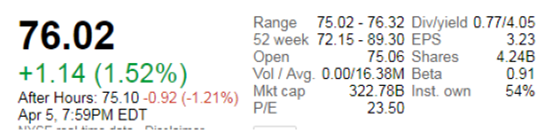Chapter 9, Problem 3TCLFundamentals of Financial Manageme...

14th Edition
Eugene F. Brigham + 1 other
ISBN: 9781285867977

Solutions

Chapter
SectionFundamentals of Financial Manageme...

14th Edition
Eugene F. Brigham + 1 other
ISBN: 9781285867977
Textbook Problem

Estimating Exxon Mobil Corporation's Intrinsic Stock ValueUse online resources to work on this chapter's questions. Please note that website information changes over time, and these changes may limit your ability to answer some of these questions.In this chapter, we described the various factors that influence stock prices and the approaches that analysts use to estimate a stock’s intrinsic value. By comparing these intrinsic value estimates to the current price, an investor can assess whether it makes sense to buy or sell a particular stock. Stocks trading at a price far below their estimated intrinsic values may be good candidates for purchase, whereas stocks trading at prices far in excess of their intrinsic value may be good stocks to avoid or sell Although estimating a stock's intrinsic value is a complex exercise that requires reliable data and good judgment, we can use the Internet to find financial data in order to arrive at a quick "back-of-the- envelope" calculation of intrinsic value.3. To provide a starting point for gauging a company's relative valuation, analysts often look at a company's price-to-earnings (P/E) ratio. Go to the website's summary quote or key statistics screen to see XOM's forward P/E ratio, which uses XOM's next 12-month estimate of earnings in the calculation, and to see its current P/E ratio. What are the firm’s forward and current P/E ratios?Summary Introduction

To identify: The P/E ratio of the given company.

Introduction:

Profit Earning Ratio (P/E Ratio): It refers to the ratio between the price of a share of a company and the earnings on the share, which is earning per share of the company. In other words, it can be defined as the ratio between the price per share of the company and its earnings per share.

Explanation

Given information:

Earnings per share are $3.23. Share price is$76.02

Formula to compute the P/E ratio:

P/E Ratio=Share PriceEarnings per Share

Substitute $3.23 for earnings per share and$76.02 for share price.

P/E Ratio=$76.02$3

Still sussing out bartleby?

Check out a sample textbook solution.

See a sample solution

The Solution to Your Study Problems

Bartleby provides explanations to thousands of textbook problems written by our experts, many with advanced degrees!

Get Started

Find more solutions based on key concepts